## Tamilnadu Samacheer Kalvi 10th Maths Solutions Chapter 3 Algebra Ex 3.17

10th Maths Exercise 3.17 Answers Question 1.
If A = $$\left[\begin{array}{cc}{1} & {9} \\ {3} & {4} \\ {8} & {-3}\end{array}\right]=\left[\begin{array}{ll}{5} & {7} \\ {3} & {3} \\ {1} & {0}\end{array}\right]$$ then verify that
(i) A + B = B + A
(ii) A + (-A) = (-A) + A = 0
Solution: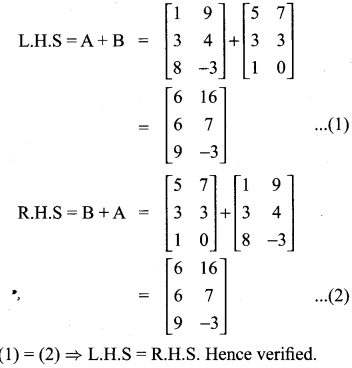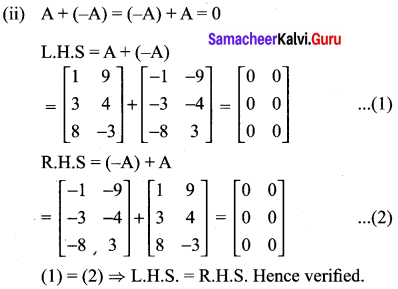Exercise 3.17 Class 10 Question 2.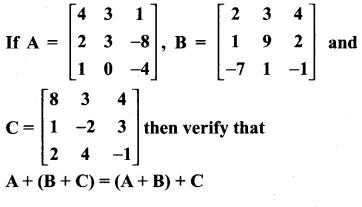Solution: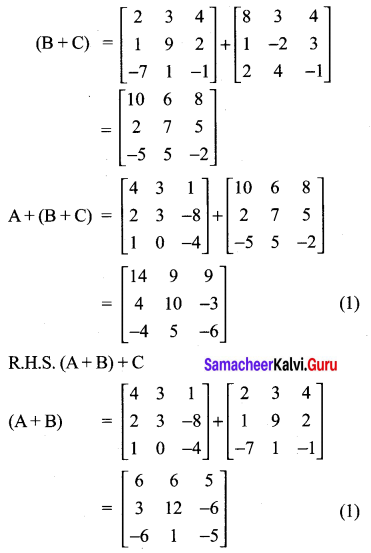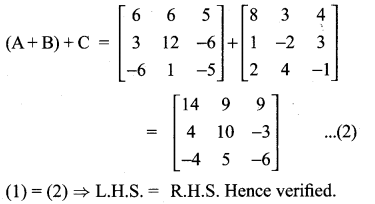Ex 3.17 Class 10 Question 3.
Find X and Y if X + Y = $$\left[\begin{array}{ll}{7} & {0} \\ {3} & {5}\end{array}\right]$$ and X – Y = $$\left[\begin{array}{ll}{3} & {0} \\ {0} & {4}\end{array}\right]$$
Solution: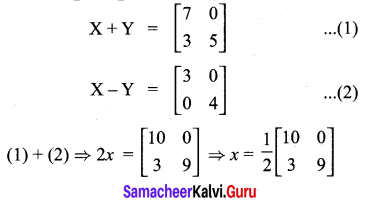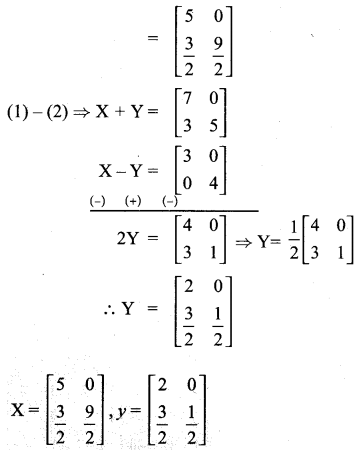10th Maths Exercise 3.17 Question 4.
If A = $$\left[\begin{array}{lll}{0} & {4} & {9} \\ {8} & {3} & {7}\end{array}\right]$$, B = $$\left[\begin{array}{lll}{7} & {3} & {8} \\ {1} & {4} & {9}\end{array}\right]$$ find the value of
(i) B – 5A
(ii) 3A – 9B
Solution: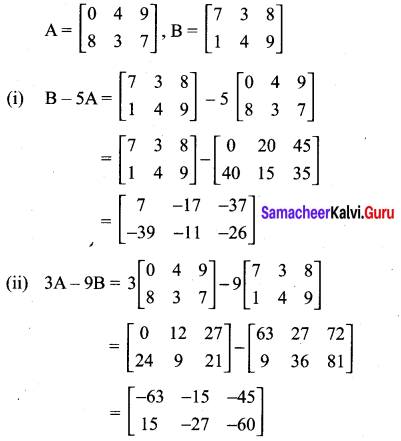10th New Syllabus Maths Exercise 3.17 Question 5.
Find the values of x, y, z if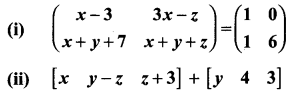Solution:
(i) $$\left(\begin{array}{cc}{x-3} & {3 x-z} \\ {x+y+7} & {x+y+z}\end{array}\right)=\left(\begin{array}{ll}{1} & {0} \\ {1} & {6}\end{array}\right)$$
x – 3 = 1 ⇒ x = 4
3x – z = 0
3(4) – z = 0
-z = -12 ⇒ z = 12
x + y + 7 = 1
x + y = -6
4 + y = -6
y = -10
x = 4, y = -10, z = 12

(ii) $$\left[\begin{array}{ccc}{x} & {y-z} & {z+3}\end{array}\right]+\left[\begin{array}{lll}{y} & {4} & {3}\end{array}\right]=\left[\begin{array}{lll}{4} & {8} & {16}\end{array}\right]$$
x + y = 4 ……………. (1)
y – z + 4 = 8 ………….. (2)
z + 3 + 3 = 16 ………….. (3)
From (3), we get z = 10
From (2), we get y – 10 + 4 = 8
From (2), we get y = 14
From (1) we get x + 14 = 4
x = -10
x = -10, y = 14, z = 10

10th Maths Exercise 3.17 Samacheer Kalvi Question 6.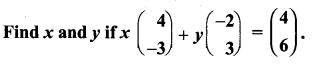Solution: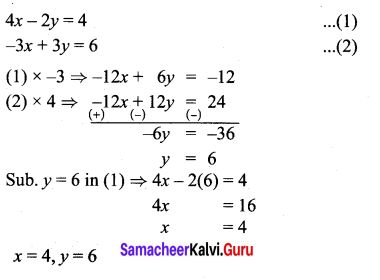10th Samacheer Maths Question 7.
Find the non-zero values of x satisfying the matrix equation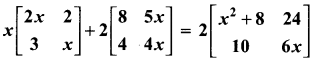Solution: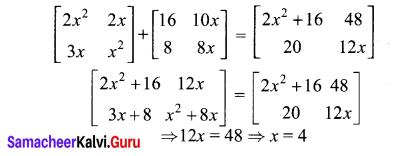Samacheer Kalvi 10th Guide Maths Question 8.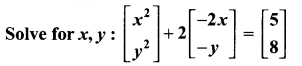Solution:
x2 – 4x = 5
y2 – 2y = 8
y2 – 2y – 8 = 0
(y – 4)(y + 2) = 0
y = 4, -2
x2 – 4x – 5 = 0
(x – 5)(x + 1) = 0
x = 5, -1
x = -1, 5, y = 4, -2